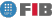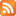# Introduction to Logic (IL)

Credits Dept. Type Requirements
7.5 (6.0 ECTS) CS-MA2
• Compulsory for DIE
• Compulsory for DCSFW
• Compulsory for DCSYS

## Instructors

 Person in charge: (-) Others: (-)

## General goals

The aim of this course is to provide a solid basis of theoretical and practical knowledge on logic for computer scientists, and, at the same time, to contribute to the students' global education in formal reasoning (on sets, relations, functions, orders, recursion and counting) and proof techniques (counter example, induction, by contradiction).

## Specific goals

### Knowledges

1. The concept of what a logic is, in terms of syntax (what is a formula F) and semantics (what is an interpretation I, and when an I satisfies an F).
2. The definition (syntax and semantics) of two logics: propositional logic and
first-order logic.
3. The concepts of tautology/validity, contradiction, logical consequence and equivalence (independently of the concrete logic under consideration), how they are used for formalizing practical problems in computer science, and how they reduce to satisfiability problems.
4. The deduction techniques for determining these properties that are most relevant in computer science: Davis-Putnam and resolution; their correctness and completeness with respect to the definition of the logic.
5. The expressive power of the two logics, and understanding in which sense propositional logic is a restriction of first-order logic; trade-off between expressiveness and good computational properties: first intuitive notions of complexity and decidability.
6. Some of the -more and more frequent- computer science applications of the deduction methods: foundations of logic programming (Prolog), deductive databases, circuits, planning problems, etc.

### Abilities

1. Understanding, writing and manipulating formulae in both logics, with special attention to computer science applications.
2. o be able to formally prove simple properties on formulae, sets, relations, functions, etc, using proof techniques such as counter examples, induction or contradiction.
3. To be able to apply resolution and Davis-Putnam to practical examples that are feasible by hand, and to know how to apply resolution as a computing mechanism (answer computation).
4. To know how to express some practica NP-complete problems (sudokus, timetabling,graph problemas, circuits) as propositional satisfiability problems, and to solve them using a SAT solver.
5. To know how to express simple problems in Prolog, and to understand Prolog's "extra-logical" extensions (its built-in arithmetic, the cut operator and its negation, its input/output).

### Competences

(no available informacion)

## Contents

Estimated time (hours):

 T P L Alt Ext. L Stu A. time Theory Problems Laboratory Other activities External Laboratory Study Additional time

1. Introduction and motivation
T      P      L      Alt    Ext. L Stu    A. time Total
2,0 0 0 0 0 2,0 0 4,0
-Importance of logic in Computer Science, examples
-Computer Science Logic vs. Mathematical Logic
-What is a logic
-Aims of this course

2. Definition of Propositional Logic
T      P      L      Alt    Ext. L Stu    A. time Total
12,0 0 0 0 0 12,0 0 24,0
-Syntax and Semantics of Propositional Logic
-Satisfaction, validity, consequence and equivalence
-All these notions can be expressed as SAT
-Expressive power: which things can be modeled
-Exercises on: induction, unique readability of formulas,
formalization of problems, counting (of formulas, interpretations, etc.),
problems on logical consequence and equivalence, intuitions on
polynomial vs. exponential computational costs (truth tables), linear
time evaluation of formulas, among others.
• Laboratory
Note: no distinction is made between theory lectures and problem sessions.

3. Deduction in Propositional Logic
T      P      L      Alt    Ext. L Stu    A. time Total
12,0 0 0 0 0 12,0 0 24,0
- Normal forms, literals and clauses.
-Davis-Putnam-Logemann-Loveland algorithm (DPLL)
(from truth tables and decision trees),
The explicit stack of Backtracking
-How to express problems in SAT and use SAT-solvers
-Intuition of NP and NP complete problems
-Deductive systems: properties of correctness, completeness and refutational completeness.
-Resolution: closure under resolution.
Exercises on normal forms, cost of the transformations,
relationship with digital circuits, deduction by resolution and DPLL, and formulation of problems in SAT: (sudokus, vertex cover, planning, hitting set)

4.
T      P      L      Alt    Ext. L Stu    A. time Total
15,0 0 0 0 0 15,0 0 30,0
-Sintax and semantics of LPO
-Closed formulas
-Expressive power and decidability
-LP as a particular case of LPO
-First-order logics with equality and theories
Exercises on: unique legibility of formulas,
formalitzation of problems, counting (of formulas, interpretations, etc.),
problems of logic consequence and equivalence,
finite and infinite models,etc.

5.
T      P      L      Alt    Ext. L Stu    A. time Total
15,0 0 0 0 0 15,0 0 30,0
-Normal forms, literals and clauses.
-Clause form transformation, Skolemitzation
-Unification, simultaneous unifier, unification rules:
termination and correctness.
-Resolution and factorization: correctness and refutational completeness
-No-termination: decision procedures and (co-)semi-decision procedures
Exercises on formalitzation, clause form transformation, unification, resolution, and (semi-)decision problems applied to programs and compilation.

6.
T      P      L      Alt    Ext. L Stu    A. time Total
14,0 0 0 0 0 14,0 0 28,0
-Answer computation: how to find the values of X that satisfy F\models \exists X G?
- SLD resolution for Horn clauses
-The backtracking stack: what does the cut operator do?
-Other extra-logic aspects: negation, predefined arithmetic,
input-output.
Exercises on resolution with answer computation, on theory and
the behavior of Prolog programs, and the implementation of Prolog programs.

 Total per kind T P L Alt Ext. L Stu A. time Total 70,0 0 0 0 0 70,0 0 140,0 Avaluation additional hours 0 Total work hours for student 140,0

## Docent Methodolgy

Theory and problems will be interleaved during the lectures (a total of five hours per week), depending on the needs at each moment. It is not convenient to rigidly define which and how many hours per week will be problem sessions. There are no lab sessions.

The methodology is based on the following principles:

1. The lecture is a starting point for the student's independent study and work.

2. As many as possible materials will be provided (lecture notes, solved exercises, unsolved ones, bibliographical references).

3. Lectures will be exploited for motivation (with examples, debates, commments, etc.) and intuition behind the applied definitions, properties and techniques will be given.

## Evaluation Methodgy

Around the week 7 of the quatrimester an exam of around 90 minutes will be taken, on the two main propositional logic chapters, with a weight of 40% of the final grade.

The final exam, which will take place during the exams period, will have two parts: on the two main propositional logic chapters (with a weight of 40%) and on
first-order logic and logic programming (60%). Any student can freely decide whether or not to do
the first part.
The propositional logic grade will be the maximum of the one obtained in the partial exam and this part of the final exam. That is, even if the grade of the partial exam is very high, there is no risk of losing it.

The exams will consist of questions on understanding of the concepts, on proving propoerties, on applying deduction techniques, and solving Prolog problems.

Any attempt of fraud during the course will entail the application of the UPC's general academic normative and the beginning of a disciplinary process.

## Basic Bibliography

• Uwe Schöning Logic for computer scientists, Birkhäuser, 1989.
• María Teresa Hortalá González, Javier Leach Albert, Mario Rodríguez Artalejo Matemática discreta y lógica matemática, Complutense, 2001.

## Complementary Bibliography

(no available informacion)© Barcelona school of informatics - Contact -RSS